## RS Aggarwal Class 8 Solutions Chapter 1 Rational Numbers Ex 1C

These Solutions are part of RS Aggarwal Solutions Class 8. Here we have given RS Aggarwal Solutions Class 8 Chapter 1 Rational Numbers Ex 1C.

Other Exercises

Question 1.
Solution:
(i) $$\\ \frac { -2 }{ 5 }$$ + $$\\ \frac { 4 }{ 5 }$$
= $$\\ \frac { -2+4 }{ 5 }$$ = $$\\ \frac { 2 }{ 5 }$$Question 2.
Solution:
(i) $$\frac { 3 }{ 4 } +\left( \frac { -3 }{ 5 } \right)$$
$$\frac { 15+\left( -12 \right) }{ 20 } =\frac { 15-12 }{ 20 } =\frac { 3 }{ 20 }$$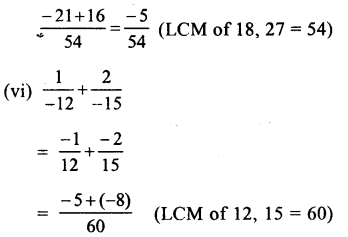Question 3.
Solution:
(i) L.H.S = $$\\ \frac { -12 }{ 5 }$$ + $$\\ \frac { 2 }{ 7 }$$
= $$\\ \frac { -84+10 }{ 35 }$$ = $$\\ \frac { -74 }{ 35 }$$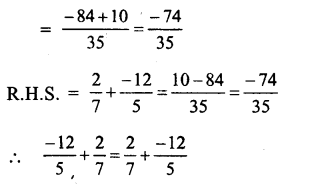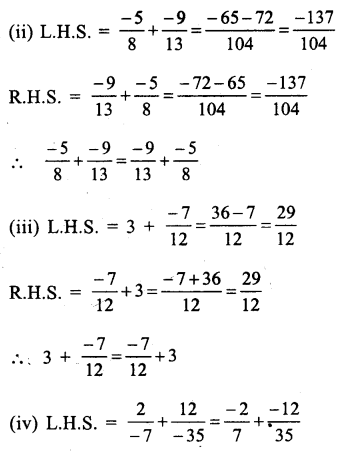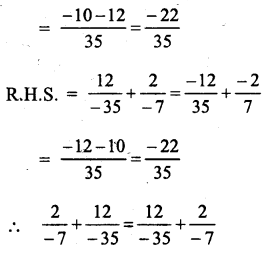Question 4.
Solution: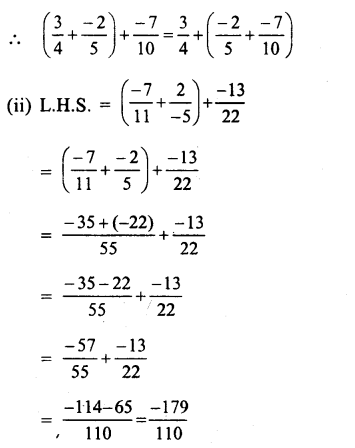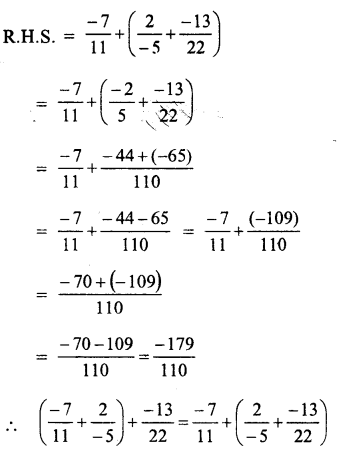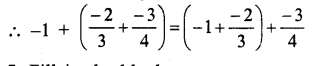Question 5.
Solution: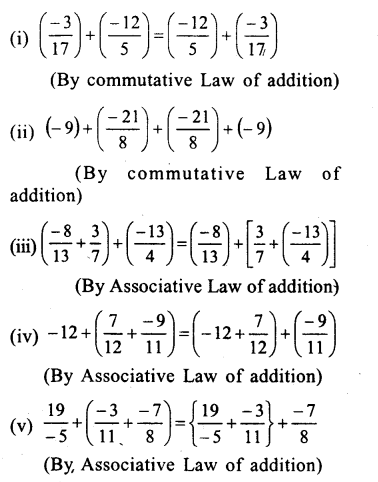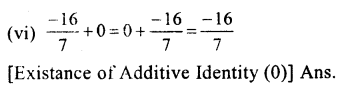Question 6.
Solution:
We know that additive inverse of $$\\ \frac { a }{ b }$$ is $$\\ \frac { -a }{ b }$$ and of $$\\ \frac { -a }{ b }$$ is $$\\ \frac { a }{ b }$$.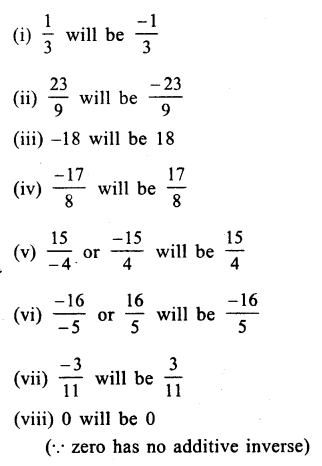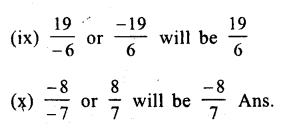Question 7.
Solution:
(i) $$\\ \frac { 3 }{ 4 }$$ from $$\\ \frac { 1 }{ 3 }$$ or $$\\ \frac { 1 }{ 3 }$$ – $$\\ \frac { 3 }{ 4 }$$
= $$\\ \frac { 4-9 }{ 12 }$$ = $$\\ \frac { -5 }{ 12 }$$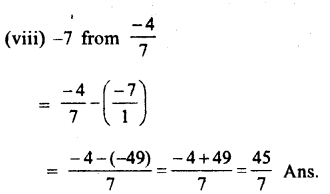Question 8.
Solution: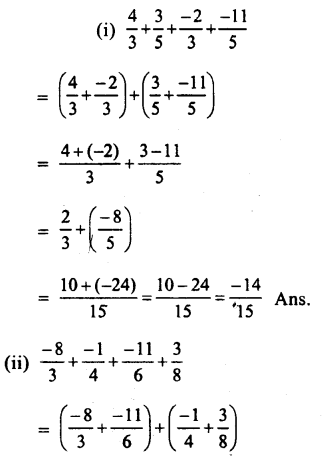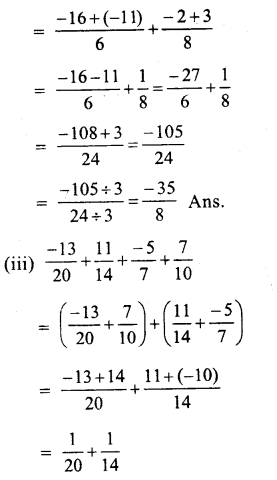Question 9.
Solution:
Sum of two numbers = – 2
one number = $$\\ \frac { -14 }{ 5 }$$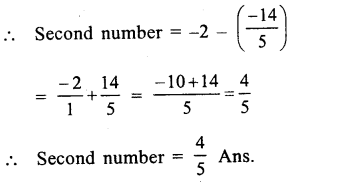Question 10.
Solution:
Sum of two numbers = $$\\ \frac { -1 }{ 2 }$$
One number = $$\\ \frac { 5 }{ 6 }$$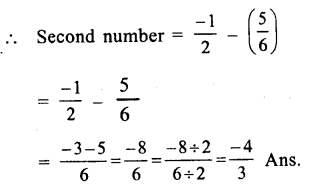Question 11.
Solution:
Sum of two numbers = $$\\ \frac { -3 }{ 2 }$$
one number = $$\\ \frac { -5 }{ 8 }$$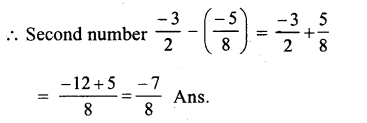Question 12.
Solution:
Sum of two numbers = $$\\ \frac { 5 }{ 7 }$$
One number = – 1
Second number = $$\\ \frac { 5 }{ 7 }$$ – ( – 1)
= $$\\ \frac { 5 }{ 7 }$$ + $$\\ \frac { 1 }{ 1 }$$
$$\\ \frac { 5+7 }{ 7 }$$ = $$\\ \frac { 12 }{ 7 }$$

Question 13.
Solution:
Difference of two numbers = $$\\ \frac { -1 }{ 6 }$$
One number = $$\\ \frac { -2 }{ 3 }$$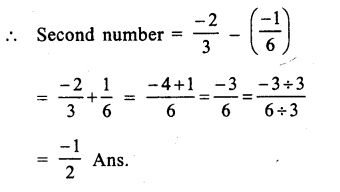Question 14.
Solution:
(i) 0 is the rational number.
(ii) Yes, the difference of two rational numbers is also rational.
(v) No, subtraction is not commutative.
(vi) No, subtraction is not associative.
(vii) The number itself.

Hope given RS Aggarwal Solutions Class 8 Chapter 1 Rational Numbers Ex 1C are helpful to complete your math homework.

If you have any doubts, please comment below. Learn Insta try to provide online math tutoring for you.

## RS Aggarwal Class 8 Solutions Chapter 1 Rational Numbers Ex 1B

These Solutions are part of RS Aggarwal Solutions Class 8. Here we have given RS Aggarwal Solutions Class 8 Chapter 1 Rational Numbers Ex 1B.

Other Exercises

Question 1.
Solution:
(i) $$\\ \frac { 1 }{ 3 }$$
Draw a line and take a point O on it.
Let it represent 0.
From O, set off unit OA, AB to right side of O, representing integers 1, 2 respectively.
Now, divide OA into 3 equal parts and take one part OR Which is $$\\ \frac { 1 }{ 3 }$$ as shown below on the line.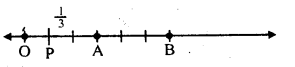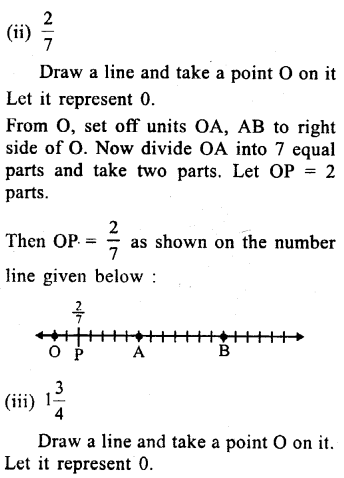Question 2.
Solution:
(i) $$\\ \frac { -1 }{ 3 }$$
Draw a line and take a point O on it.
Let it represent O.
Now, from O, take OA, AB to the left of O, representing integers – 1, – 2 respectively. Divide OA into three equal parts and take one part.
Then OP = $$\\ \frac { -1 }{ 3 }$$ which is shown on the number line as given below :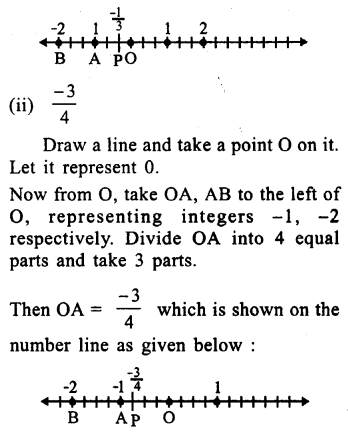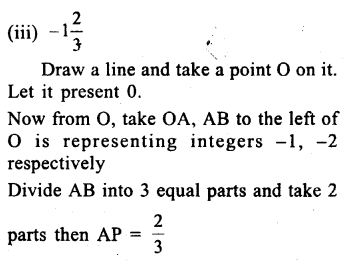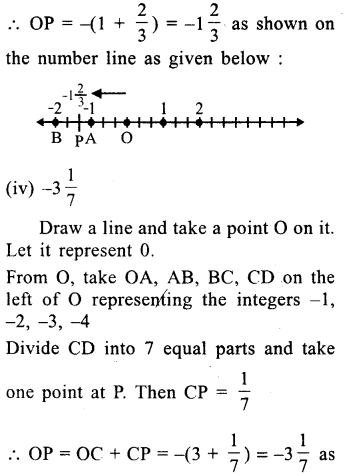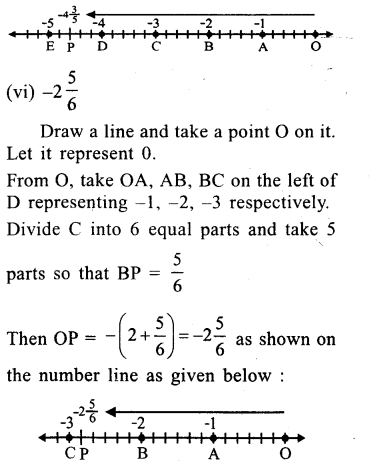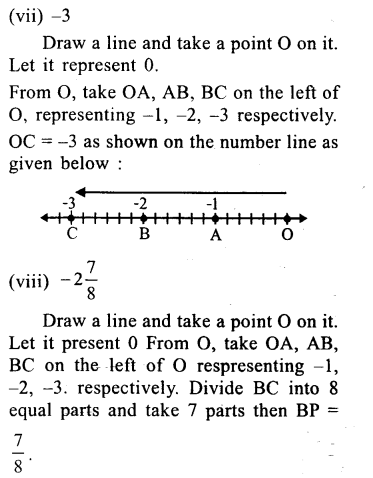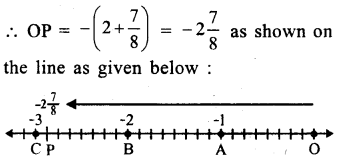Question 3.
Solution:
(i) True, as the numbers left of O are negative.
(ii) False, as the numbers right of O are positive and $$\\ \frac { -12 }{ 7 }$$ is negative.
(iii) True, as $$\\ \frac { 1 }{ 3 }$$ is positive and $$\\ \frac { -5 }{ 2 }$$ is negative.
(iv) False $$\\ \frac { -18 }{ -13 }$$ = $$\\ \frac { 18 }{ 13 }$$ which is positive and positive numbers lie on the right of O.

Hope given RS Aggarwal Solutions Class 8 Chapter 1 Rational Numbers Ex 1B are helpful to complete your math homework.

If you have any doubts, please comment below. Learn Insta try to provide online math tutoring for you.

## Online Education for RS Aggarwal Class 8 Solutions Chapter 11 Compound Interest Ex 11C

These Solutions are part of Online Education RS Aggarwal Solutions Class 8. Here we have given RS Aggarwal Solutions Class 8 Chapter 11 Compound Interest Ex 11C.

Other Exercises

Question 1.
Solution:
Principal (P) = Rs. 8000
Rate (R) = 10% p.a. or 5% half yearly
Period (n) = 1 year or 2 half years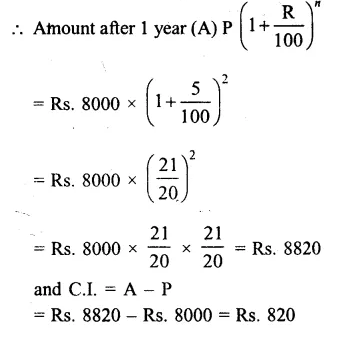Question 2.
Solution:
Principal (P) = Rs. 31250
Rate (R) = 8% p.a. or 4% half yearly
Period (n) = $$1\frac { 1 }{ 2 }$$ years or 3 half years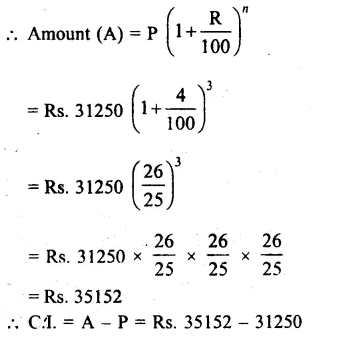= Rs 3902

Question 3.
Solution:
Principal (P) = Rs 12800
Rate (R) = $$7\frac { 1 }{ 2 }$$% p.a. = $$\\ \frac { 15 }{ 2 }$$% half yearly
Period (n) = 1 year or 2 half years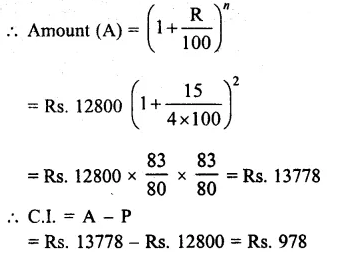Question 4.
Solution:
Principal (P) = Rs. 160000
Rate (R) = 10% p.a. or 5% half yearly
Period (n) = 2 years or 4 half years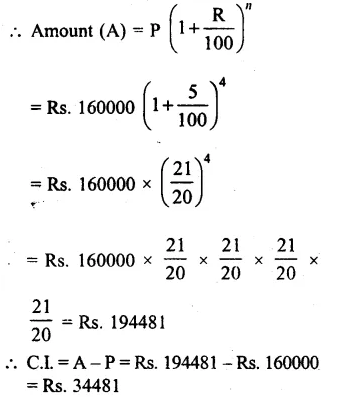Question 5.
Solution:
Principal (P) = Rs. 40960
Rate (R) = $$12\frac { 1 }{ 2 }$$ = $$\\ \frac { 25 }{ 2 }$$% p.a. or $$\\ \frac { 25 }{ 4 }$$ % half yearlyQuestion 6.
Solution:
Loan received for the cost of the house (P) = Rs. 125000
Rate of interest (R) = 12% p.a. or 6% half yearlyQuestion 7.
Solution:
Amount deposit in the bank = Rs. 20000
Rate of interest (R) = 6% p.a. or 3% half-yearly
Period (n) = 1 year or 2 half years
= $${ \left( 1+\frac { R }{ 100 } \right) }^{ n }$$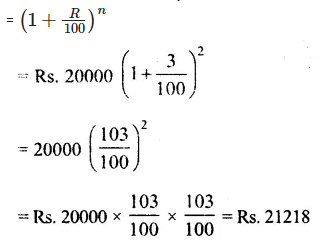Question 8.
Solution:
Amount of loan = Rs. 65536
Rate of interest (R) = 12 $$12\frac { 1 }{ 2 }$$ % = $$\\ \frac { 25 }{ 2 }$$%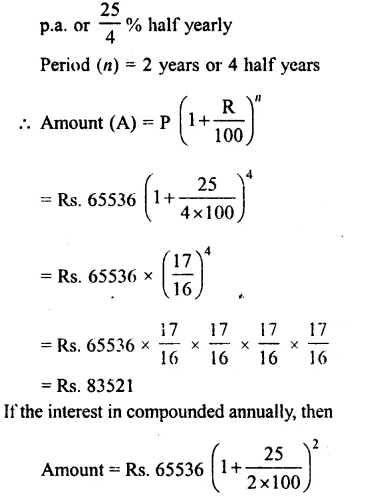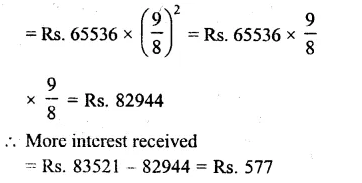Question 9.
Solution:
Amount deposit in the bank (P)
= Rs. 32000
Rate of interest (R) = 5% p.a.Question 10.
Solution:
Amount taken from finance company (P) = Rs. 390625
Rate of interest (R) = 16% p.a.Hope given RS Aggarwal Solutions Class 8 Chapter 11 Compound Interest Ex 11C are helpful to complete your math homework.

If you have any doubts, please comment below. Learn Insta try to provide online math tutoring for you.